# Math 1050 - MAPLE LAB III

Maple Lab III

## Math 1050 - MAPLE LAB 3

Exponential and Logarithmic Functions:
Exponential Functions

Exponential functions are of the form f(x)=bx, where x is the exponent and b the base. This basic form of an exponential function creates two general shapes in graphs. Let's look at the graphs of f(x)=5x, g(x)=2x, h(x)= (1/2)x, and j(x)=(1/3)x   to see what these shapes are. Remember that we can name these functions as f, g, h and j, or we can just use their algebraic expression to graph them. To display both methods I will graph the first two by defining them with their names and the second two by typing the algebraic expression directly into to plot command.

Remember to be careful when defining functions; type the function name:=variable->expression. Each piece is essential.

> f:=x->5^x;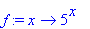> g:=x->2^x;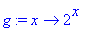> plot(f(x),x=-5..5,y=-5..5);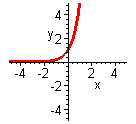> plot(g(x),x=-5..5,y=-5..5);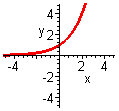> plot((1/2)^x,x=-5..5,y=-5..5);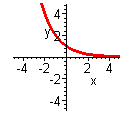> plot((1/3)^x,x=-5..5,y=-5..5);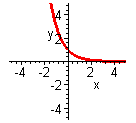What similarities do these graphs share? What patterns start to arise? The graphs of f and g are both increasing everywhere while the graphs of h and j are decreasing everywhere. All of the graphs cross the y axis at 1, and none of them touch or cross the x axis.
Conclusion: for the graph of f(x)=bx, if b>1 the graph has a y intercept of 1, and will be increasing, and if 0
If we graph g and h on the same axis, can you find any special relationship between them?

> plot({g(x),(1/2)^x},x=-5..5,y=-2..5);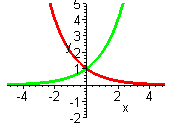It appears that they are reflections about the y axis, let's prove this is true. If this is true then it is known that h(x) and g(-x) should be equal. Try this, does g(-x)=h(x)? If g(x)=2x, then g(-x)=2-x = (2-1)x = (1/2)x =h(x), so yes the graphs are reflections of each other. In fact, two exponential functions whose bases are reciprocals will be reflections across the y axis.

Logarithmic Functions

Logarithmic functions are of the form f(x)=logb(x), where x is the input (argument) and b is the base. This basic form of a logarithmic function also creates two general shapes in graphing. Again, let's graph a couple of functions to be compared. Graph k(x)=log5(x), m(x)=log2(x), n(x)=log1/2(x), and p(x)=log1/3(x). I will define only k and m with names, then graph k and m using the name and n and p by using the algebraic form in the plot command.
To enter logarithmic function into MAPLE type log[base](argument).

> k:=x->log(x);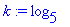> m:=x->log(x);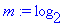Notice that the x does not show up in the function output, this is because the log is a special command in MAPLE so the x in understood, but not printed.
And all function manipulation remains the same. For instance evaluating k at x=2, as done below.

> k(2);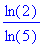Notice that MAPLE uses the conversion identity of loga(x)=ln(x)/ln(a), in order to calculate logarithmic bases other than 10 or e. In order to evaluate this as a decimal we use the evalf command.

> evalf(k(2));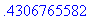> plot(k(x),x=-5..5,y=-5..5);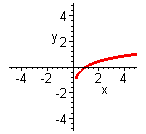> plot(m(x),x=-5..5,y=-5..5);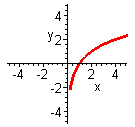> plot(log[1/2](x),x=-5..5,y=-5..5);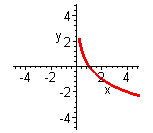> plot(log[1/3](x),x=-5..5,y=-5..5);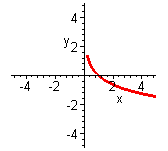What similarities do these graphs share? What patterns start to arise? The graphs of k and m are both increasing everywhere while the graphs of n and p are decreasing everywhere. All of the graphs cross the x axis at 1, and none of them touch or cross the y axis.
Conclusion: for the graph of f(x)=
logb(x), if b>1 the graph has an x intercept of 1, and will be increasing, and if 0
If we graph m and n on the same axis, can you find any special relationship between them?

> plot({m(x), log[1/2](x)},x=-5..5,y=-5..5);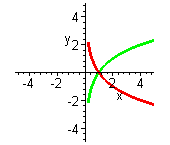It appears that they are reflections about the x axis, to prove this we'll check and see if m(x)= -n(x). If n(x)=log1/2(x), then -n(x)= -log1/2(x)  = log1/2(x-1). Using the equivalent exponential definition, if log1/2(x-1) =y, then (1/2)y =1/x, so(1/2y) =1/x, therefore 2y =x. Although, 2y =x is true if and only if log2(x) =y=m(x), so m(x)= -n(x). Therefore, yes the graphs are just reflections of each other across the x axis. In fact the graphs of two log functions that have reciprocal bases will be reflections across the x axis.

Now let's graph a comparison of the exponential and logarithmic functions 2x and log2(x) on the same axis.

> plot({2^x,log(x)},x=-5..5,y=-5..5);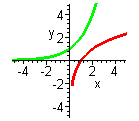What do you notice about the graphs? They appear to be reflected about the line y=x which would imply that they are inverses. Let's check and see, what is the inverse of 2x? Start with y=2x, then interchange x & y to obtain x=2y  and solve for y. If x=2y, then log2(x) = log2(2y) =y, so yes if f(x)=2x, then f-1(x) =log2(x).

What about ex and ln(x), how do their graphs compare? We know ex and ln(x) inverses, so they should be reflections about the line y=x. I will also have MAPLE plot the line y=x with the other two functions on the axis so that it is easier to compare the graphs and see that they are reflections about the line y=x. To get all three graphs to print, again I must specify the colors for MAPLE to use, otherwise it will use yellow which does not print clearly. Also notice that the command for raising e to any exponent is exp(exponent).

> plot({exp(x),ln(x),x},x=-5..5,y=-5..5, color=[red,blue,green]);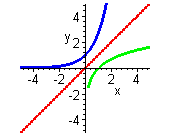Note: there is no way to have MAPLE find the inverse of f(x) using the inverse notation f-1(x) . The inverse must first be found by hand and then entered into MAPLE.

Now that we have an idea about general exponential and logarithmic functions, let's use MAPLE to graph some other nonstandard exponential and logarithmic functions. Graph 32x+5+6 and 4log9(3x-7)-4 on the same axis.

> plot({3^(2*x+5)+6,4*log(3*x+7)-4},x=-10..10,y=-10..10);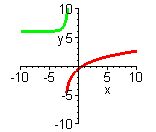The top one will be the exponential graph since as x grows, y is growing very rapidly, and the bottom one the graph of a logarithmic function.

Now look at the graph of log(x2).

> plot (log(x^2), x=-10..10,y=-10..10);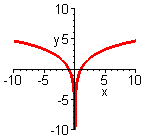This graph exists for both positive and negative x values since x is squared before the log is taken, but notice the y axis still forms an asymptote. The domain for this function would be all reals except zero.

Exercises: (For all the exercises below, display the intervals -10 <x < 10, and -10 < y < 10 on the axis)

1. For each of parts a-d, prepare a graph showing the line y=x, the function and its inverse all on the same axis. You should have one graph for each part a-d, each with three lines appearing. Note - you must find the inverse by hand, then ask MAPLE to plot it. After you print your lab, label each function on each graph by hand.
A. f(x)=3x
B. g(x)=log8(x)
C. h(x)=(1/6)x
D. j(x)=log1/5(x)

2. Graph each of the following functions on a separate axis. Then find and state the domain, range, and any vertical or horizontal asymptotes. The computer cannot do this for you, you will have to find them yourself by hand.
A.log4(x-3)
B.  log1/2(x)-6
C.  (1/2)x+5

3. Graph the functionslog3(x), log3(2x) and 2log3(x) on the same axis, label them and write how the last two graphs have changed in relation to the first.